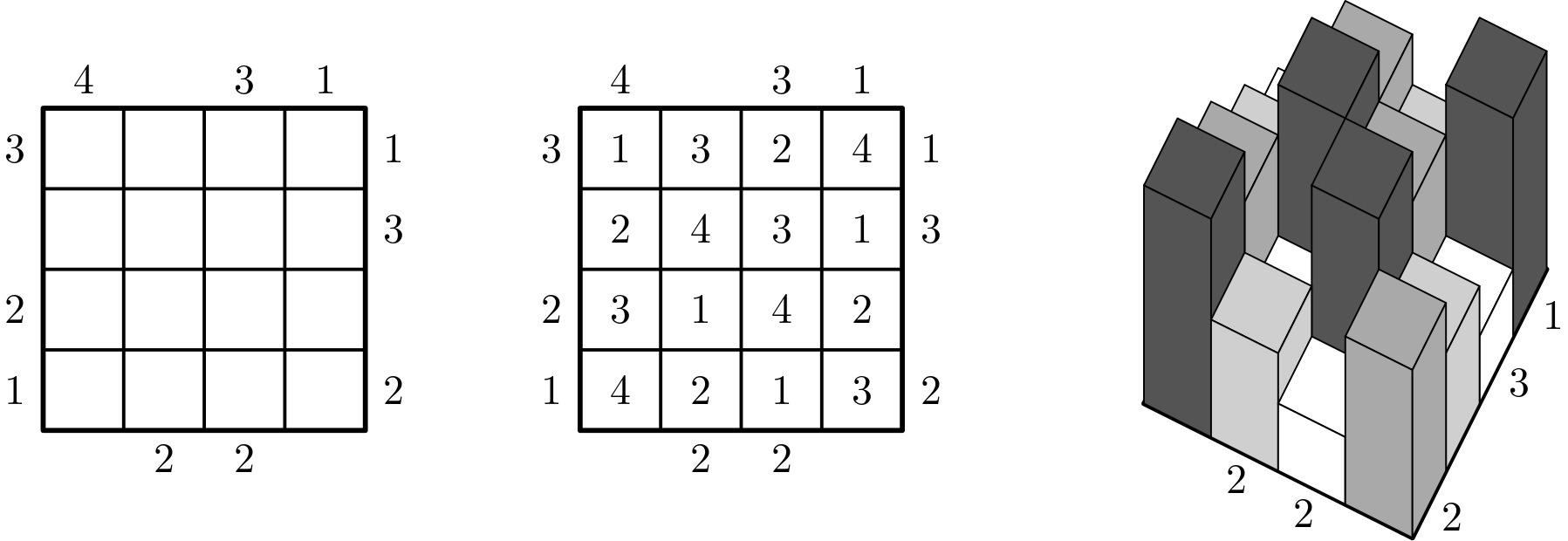# Skyscrapers (Building puzzle)

A single player game in which the player must arrange skyscrapers on a square grid so that only certain number of skyscrapers are visible from each row/column, and the skyscrapers' heights form a Latin square.## Description

Skyscrapers is a single player game played on a $n \times n$ grid. Initially each cell of the grid is empty, but some hints are given on the border of the grid. More precisely hints are natural numbers between $1$ and $n$ and can appear before the first cell of a row, after the last cell of a row, before the first cell of a column, or after the first cell of a column. The goal is that of filling the grid by writing, in each generic cell of coordinates $(i,j)$, a number $x_{i,j} \in \{1, \dots, n\}$ so that:

• The resulting grid is a Latin square, i.e., each row and each column contain all number from $1$ to $n$.
• If hint $h$ appears before the first cell (resp. after the last cell) of row $i$, then there are exactly $h$ indices $j \in \{1, \dots, n\}$ such that $x_{i,j} = \max \{x_{i,k} \mid k = 1, \dots, j \}$ (resp. $x_{i,j} = \max \{x_{i,k} \mid k = j, \dots, n \}$).
• If hint $h$ appears before the first cell (resp. after the last cell) of column $j$, then there are exactly $h$ indices $i \in \{1, \dots, n\}$ such that $x_{i,j} = \max \{x_{k,j} \mid k = 1, \dots, i \}$ (resp. $x_{i,j} = \max \{x_{k,j} \mid k = i, \dots, n \}$).

The game as a geometric interpretation: writing $x_{i,j}$ in the cell of coordinates $(i,j)$ represents building a skyscraper of height $x_{i,j}$ in that location. Only one skyscraper of a given height is allowed per row/column. Moreover, if an observer is sitting in the location of an hint $h$ and they look at the corresponding row/column, then the number of visible skyscrapers needs to be exactly $h$, where a skyscraper of a certain height hides all shorter skyscrapers behind it along the considered direction.

The left figure shows an instance of Skyscrapers. The middle figure shows a valid solution. The right figure shows a 3D view of the solution.

## Computational complexity

Given a Skyscrapers instance in which some cells of the grid have already been filled, it is NP-Complete to determine whether the grid can be completed into a valid solution .

The same holds if the instance has only one hint $h$, on the left side of the first row, some cells of the grid have already been filled, and the problem is that of determining whether the empty cells in the first row can be satisfied so that: (i) each integer in $\{1, \dots, n\}$ appears exactly once on the first row, and at most once in each column, and (ii) the hint matches the number of skyscrapers visible from the left side of the first row, i.e., there are exactly $h$ indices $j \in \{1, \dots, n\}$ such that $x_{1,j} = \max \{x_{1,k} \mid k = 1, \dots, j \}$ .

 C. Iwamoto, Y. Matsui, “Computational Complexity of Building Puzzles”, IEICE Transactions on Fundamentals of Electronics, Communications and Computer Sciences, 2016.

 K. Haraguchi, R. Tanaka, “The Building Puzzle Is Still Hard Even in the Single Lined Version”, Journal of Information Processing, 2017.

@misc{cog:skyscrapers,
author = "{CoG contributors}",
title  = "{Skyscrapers (Building puzzle) --- Complexity of Games}",
year   = "2022",
url    = "https://www.isnphard.com/i/skyscrapers/",
note   = "[Online; accessed 2022-06-02]"
}